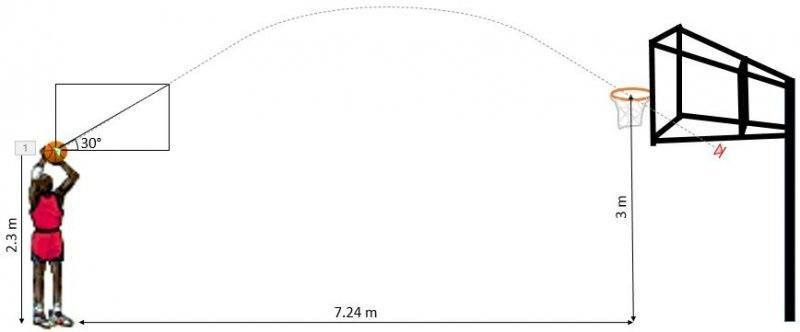# Help me calculate the speed of the basketball

Archit
Here is the picture for you to understand it better.
Please help me calculate the velocity required for the ball.
I have already tried it with the formula:
displacement = initial velocity(time) + (acceleration)(time2)/2
but cannot since I do not know the initial velocity and the time## Answers and Replies

barefeet
but cannot since I do not know the initial velocity and the time

The initial velocity is what you are looking for

Archit
The initial velocity is what you are looking for
Yes I am looking for the initial velocity. Then I will calculate the force required to throw the ball

barefeet
Start by looking at x and y components of the velocity separately. Try to figure out what equations apply to these components

Archit
Start by looking at x and y components of the velocity separately. Try to figure out what equations apply to these components
Thanks. I got my answer!